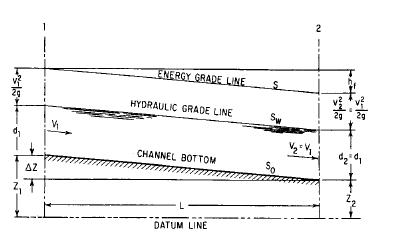Search

# Open Channel Flow

Posted in Hydraulics |Email This Post |

The flow in the pipe is said to be open channel if the pipe is only half full eg Free surface flow, or open-channel flow, includes all cases of flow in which the liquid surface is open to the atmosphere.

The discharge Q at any section is defined as the volume of water passing that section per unit of time. It is expressed in cubic feet per second, ft3/s (cubic meter per second, m3/s), and is given by

Q=VA

where V =average velocity, ft/s (m/s)

A =cross-sectional area of flow, ft2 (m2)

When the discharge is constant, the flow is said to be continuous and therefore

Q=V1 A1=V2 A2

where the subscripts designate different channel sections. This equation is known as the continuity equation for continuous steady flow.

Depth of flow d is taken as the vertical distance, ft (m), from the bottom of a channel to the water surface.
The wetted perimeter is the length, ft (m), of a line bounding the cross-sectional area of flow minus the free surface width.
The hydraulic radius R equals the area of flow divided by its wetted perimeter.
The average velocity of flow V is defined as the discharge divided by the area of flow:

V= Q/A

The velocity head HV, ft (m), is generally given by

HV=V2/2g

where V =average velocity, ft/s (m /s)

g=acceleration due to gravity, 32.2 ft/s2 (9.81 m/s2).

The true velocity head may be expressed as

H=kV2/2g

where
k is an empirical coefficient that represents the degree of turbulence. Experimental data indicate that k may vary from about 1.03 to 1.36 for prismatic channels. It is, however, normally taken as 1.00 for practical hydraulic work and is evaluated only for precise investigations of energy loss. The total energy per pound (kilogram) of water relative to the bottom of the channel at a vertical section is called the specific energy head He. It is composed of the depth of flow at any point, plus the velocity head at the point. It is
expressed in feet (meter) as

Hd=d+V2/2g

A longitudinal profile of the elevation of the specific energy head is called the energy grade line, or the total-head line

.
A longitudinal profile of the water surface is called the hydraulic grade line. The vertical distance between these profiles at any point equals the velocity head at that point.More Entries :